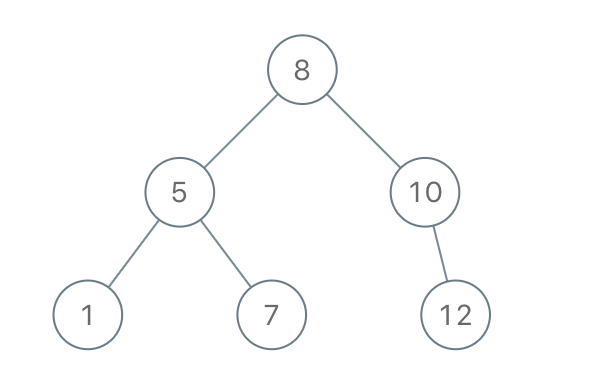## C# || How To Construct A Binary Search Tree From Preorder Traversal Using C#The following is a module with functions which demonstrates how to construct a binary search tree from preorder traversal using C#.

1. Binary Tree From Preorder – Problem Statement

Given an array of integers preorder, which represents the preorder traversal of a BST (i.e., binary search tree), construct the tree and return its root.

It is guaranteed that there is always possible to find a binary search tree with the given requirements for the given test cases.

A binary search tree is a binary tree where for every node, any descendant of Node.left has a value strictly less than Node.val, and any descendant of Node.right has a value strictly greater than Node.val.

A preorder traversal of a binary tree displays the value of the node first, then traverses Node.left, then traverses Node.right.

Example 1:``` Input: preorder = [8,5,1,7,10,12] Output: [8,5,10,1,7,null,12] ```

Example 2:

``` Input: preorder = [1,3] Output: [1,null,3] ```

2. Binary Tree From Preorder – Solution

The following is a solution which demonstrates how to construct a binary search tree from preorder traversal.

``` 2. Binary Tree From Preorder - Solution C# // ============================================================================ // Author: Kenneth Perkins // Date: Oct 16, 2021 // Taken From: http://programmingnotes.org/ // File: Solution.cs // Description: Demonstrates how to construct a BST from preorder traversal // ============================================================================ /** * Definition for a binary tree node. * public class TreeNode { * public int val; * public TreeNode left; * public TreeNode right; * public TreeNode(int val=0, TreeNode left=null, TreeNode right=null) { * this.val = val; * this.left = left; * this.right = right; * } * } */ public class Solution { private int currentIndex = 0; public TreeNode BstFromPreorder(int[] preorder) { return BuildBST(preorder, int.MaxValue); } TreeNode BuildBST(int[] preorder, int parentValue) { if (currentIndex == preorder.Length || preorder[currentIndex] > parentValue) { return null; } var currentValue = preorder[currentIndex++]; var node = new TreeNode(currentValue); node.left = BuildBST(preorder, currentValue); node.right = BuildBST(preorder, parentValue); return node; } }// http://programmingnotes.org/ 123456789101112131415161718192021222324252627282930313233343536373839 // ============================================================================//    Author: Kenneth Perkins//    Date:   Oct 16, 2021//    Taken From: http://programmingnotes.org///    File:  Solution.cs//    Description: Demonstrates how to construct a BST from preorder traversal// ============================================================================/** * Definition for a binary tree node. * public class TreeNode { *     public int val; *     public TreeNode left; *     public TreeNode right; *     public TreeNode(int val=0, TreeNode left=null, TreeNode right=null) { *         this.val = val; *         this.left = left; *         this.right = right; *     } * } */public class Solution {    private int currentIndex = 0;     public TreeNode BstFromPreorder(int[] preorder) {        return BuildBST(preorder, int.MaxValue);    }     TreeNode BuildBST(int[] preorder, int parentValue) {        if (currentIndex == preorder.Length || preorder[currentIndex] > parentValue) {            return null;        }         var currentValue = preorder[currentIndex++];        var node = new TreeNode(currentValue);        node.left = BuildBST(preorder, currentValue);        node.right = BuildBST(preorder, parentValue);        return node;    }}// http://programmingnotes.org/ ```

QUICK NOTES:
The highlighted lines are sections of interest to look out for.

The code is heavily commented, so no further insight is necessary. If you have any questions, feel free to leave a comment below.

Once compiled, you should get this as your output for the example cases:

``` [8,5,10,1,7,null,12] [1,null,3] ```